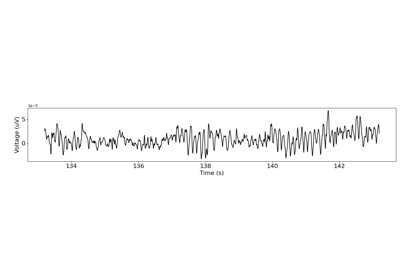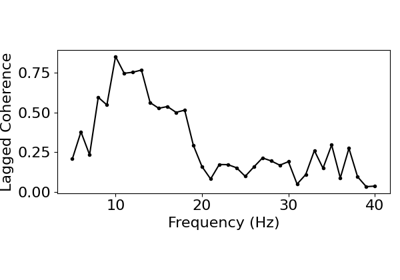# neurodsp.rhythm.compute_lagged_coherence¶

neurodsp.rhythm.compute_lagged_coherence(sig, fs, freqs, n_cycles=3, return_spectrum=False)[source]

Compute lagged coherence, reflecting the rhythmicity across a frequency range.

Parameters
sig1d array

Time series.

fsfloat

Sampling rate, in Hz.

freqs1d array or list of float

If array, frequency values to estimate with morlet wavelets. If list, define the frequency range, as [freq_start, freq_stop, freq_step]. The freq_step is optional, and defaults to 1. Range is inclusive of freq_stop value.

n_cyclesfloat or list of float, default: 3

Number of cycles of each frequency to use to compute lagged coherence. If a single value, the same number of cycles is used for each frequency value. If a list or list_like, then should be a n_cycles corresponding to each frequency.

return_spectrumbool, optional, default: False

If True, return the lagged coherence for all frequency values. Otherwise, only the mean lagged coherence value across the frequency range is returned.

Returns
lcsfloat or 1d array

If return_spectrum is False: mean lagged coherence value across the frequency range. If return_spectrum is True: lagged coherence values for all frequencies.

freqs1d array

Frequencies, corresponding to the lagged coherence values, in Hz. Only returned if return_spectrum is True.

References

1

Fransen, A. M., van Ede, F., & Maris, E. (2015). Identifying neuronal oscillations using rhythmicity. Neuroimage, 118, 256-267. DOI: 10.1016/j.neuroimage.2015.06.003

Examples

Compute lagged coherence for a simulated signal with beta oscillations:

>>> from neurodsp.sim import sim_combined
>>> sig = sim_combined(n_seconds=10, fs=500,
...                    components={'sim_synaptic_current': {},
...                                'sim_bursty_oscillation': {'freq': 20,
...                                                           'enter_burst': .50,
...                                                           'leave_burst': .25}})
>>> lag_cohs = compute_lagged_coherence(sig, fs=500, freqs=(5, 35))


## Examples using neurodsp.rhythm.compute_lagged_coherence¶